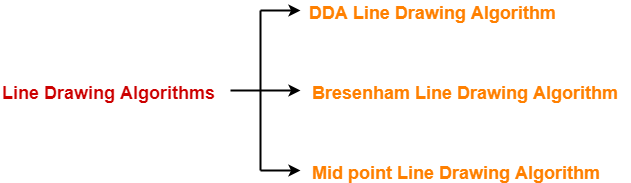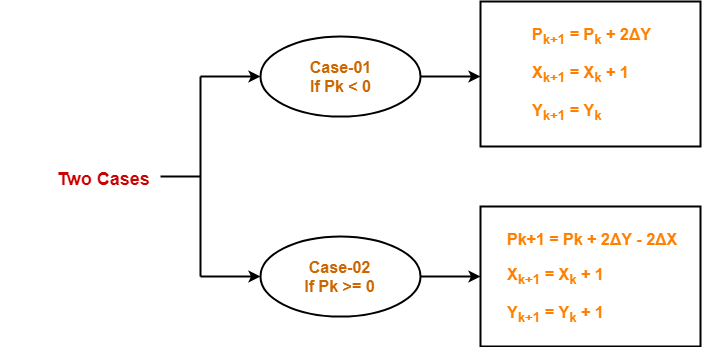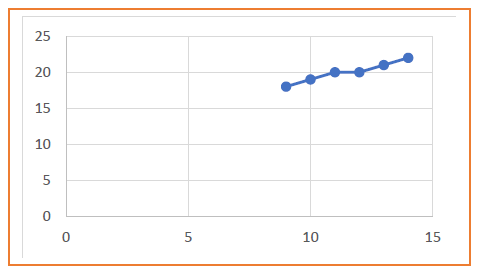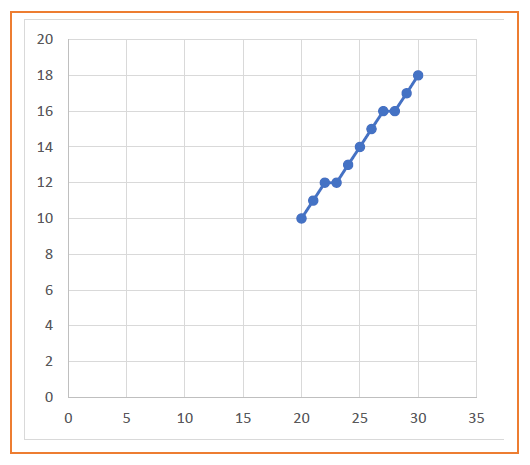## Line Drawing Algorithms-

In computer graphics, popular algorithms used to generate lines are-1. Digital Differential Analyzer (DDA) Line Drawing Algorithm
2. Bresenham Line Drawing Algorithm
3. Mid Point Line Drawing Algorithm

## Bresenham Line Drawing Algorithm-

 Given the starting and ending coordinates of a line,Bresenham Line Drawing Algorithm attempts to generate the points between the starting and ending coordinates.

### Procedure-

Given-

• Starting coordinates = (X0, Y0)
• Ending coordinates = (Xn, Yn)

The points generation using Bresenham Line Drawing Algorithm involves the following steps-

### Step-01:

Calculate ΔX and ΔY from the given input.

These parameters are calculated as-

• ΔX = Xn – X0
• ΔY =Yn – Y0

### Step-02:

Calculate the decision parameter Pk.

It is calculated as-

Pk = 2ΔY – ΔX

### Step-03:

Suppose the current point is (Xk, Yk) and the next point is (Xk+1, Yk+1).

Find the next point depending on the value of decision parameter Pk.### Step-04:

Keep repeating Step-03 until the end point is reached or number of iterations equals to (ΔX-1) times.

## Problem-01:

Calculate the points between the starting coordinates (9, 18) and ending coordinates (14, 22).

## Solution-

Given-

• Starting coordinates = (X0, Y0) = (9, 18)
• Ending coordinates = (Xn, Yn) = (14, 22)

### Step-01:

Calculate ΔX and ΔY from the given input.

• ΔX = Xn – X0 = 14 – 9 = 5
• ΔY =Yn – Y0 = 22 – 18 = 4

### Step-02:

Calculate the decision parameter.

Pk

= 2ΔY – ΔX

= 2 x 4 – 5

= 3

So, decision parameter Pk = 3

### Step-03:

As Pk >= 0, so case-02 is satisfied.

Thus,

• Pk+1 = Pk + 2ΔY – 2ΔX = 3 + (2 x 4) – (2 x 5) = 1
• Xk+1 = Xk + 1 = 9 + 1 = 10
• Yk+1 = Yk + 1 = 18 + 1 = 19

Similarly, Step-03 is executed until the end point is reached or number of iterations equals to 4 times.

(Number of iterations = ΔX – 1 = 5 – 1 = 4)

 Pk Pk+1 Xk+1 Yk+1 9 18 3 1 10 19 1 -1 11 20 -1 7 12 20 7 5 13 21 5 3 14 22## Problem-02:

Calculate the points between the starting coordinates (20, 10) and ending coordinates (30, 18).

## Solution-

Given-

• Starting coordinates = (X0, Y0) = (20, 10)
• Ending coordinates = (Xn, Yn) = (30, 18)

### Step-01:

Calculate ΔX and ΔY from the given input.

• ΔX = Xn – X0 = 30 – 20 = 10
• ΔY =Yn – Y0 = 18 – 10 = 8

### Step-02:

Calculate the decision parameter.

Pk

= 2ΔY – ΔX

= 2 x 8 – 10

= 6

So, decision parameter Pk = 6

### Step-03:

As Pk >= 0, so case-02 is satisfied.

Thus,

• Pk+1 = Pk + 2ΔY – 2ΔX = 6 + (2 x 8) – (2 x 10) = 2
• Xk+1 = Xk + 1 = 20 + 1 = 21
• Yk+1 = Yk + 1 = 10 + 1 = 11

Similarly, Step-03 is executed until the end point is reached or number of iterations equals to 9 times.

(Number of iterations = ΔX – 1 = 10 – 1 = 9)

 Pk Pk+1 Xk+1 Yk+1 20 10 6 2 21 11 2 -2 22 12 -2 14 23 12 14 10 24 13 10 6 25 14 6 2 26 15 2 -2 27 16 -2 14 28 16 14 10 29 17 10 6 30 18## Advantages of Bresenham Line Drawing Algorithm-

The advantages of Bresenham Line Drawing Algorithm are-

• It is easy to implement.
• It is fast and incremental.
• It executes fast but less faster than DDA Algorithm.
• The points generated by this algorithm are more accurate than DDA Algorithm.
• It uses fixed points only.

## Disadvantages of Bresenham Line Drawing Algorithm-

The disadvantages of Bresenham Line Drawing Algorithm are-

• Though it improves the accuracy of generated points but still the resulted line is not smooth.
• This algorithm is for the basic line drawing.
• It can not handle diminishing jaggies.

To gain better understanding about Bresenham Line Drawing Algorithm,

Watch this Video Lecture

Next Article-Mid Point Line Drawing Algorithm

Get more notes and other study material of Computer Graphics.

Watch video lectures by visiting our YouTube channel LearnVidFun.